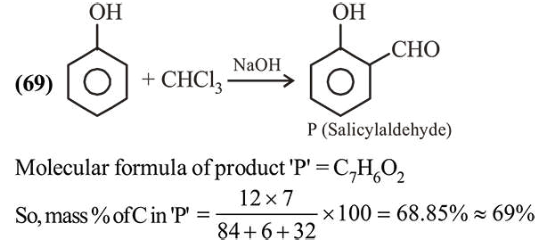# A solution of phenol in chloroform when treated with aqueous

Question:

A solution of phenol in chloroform when treated with aqueous $\mathrm{NaOH}$ gives compound $\mathrm{P}$ as a major product. The mass percentage of carbon in $\mathrm{P}$ is (to the nearest integer) (Atomic mass: $\mathrm{C}=12 ; \mathrm{H}=1 ; \mathrm{O}=16$ )

Solution: